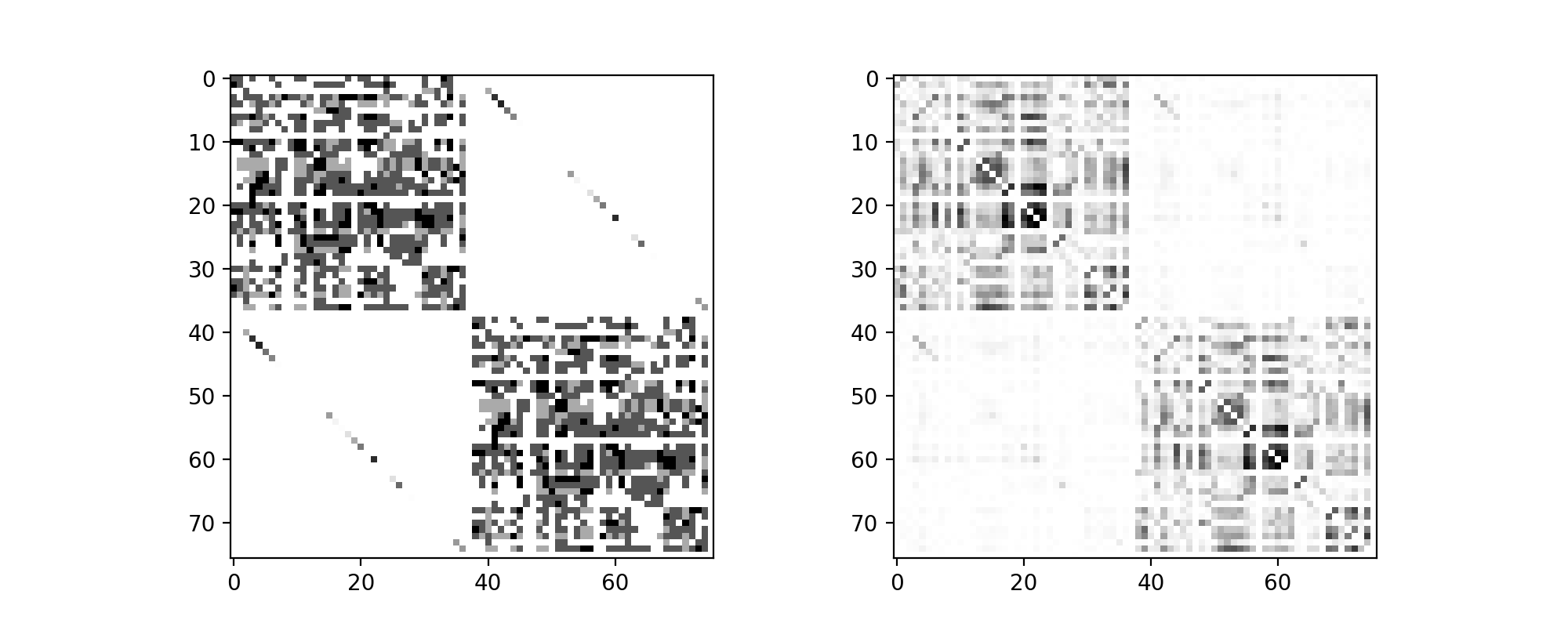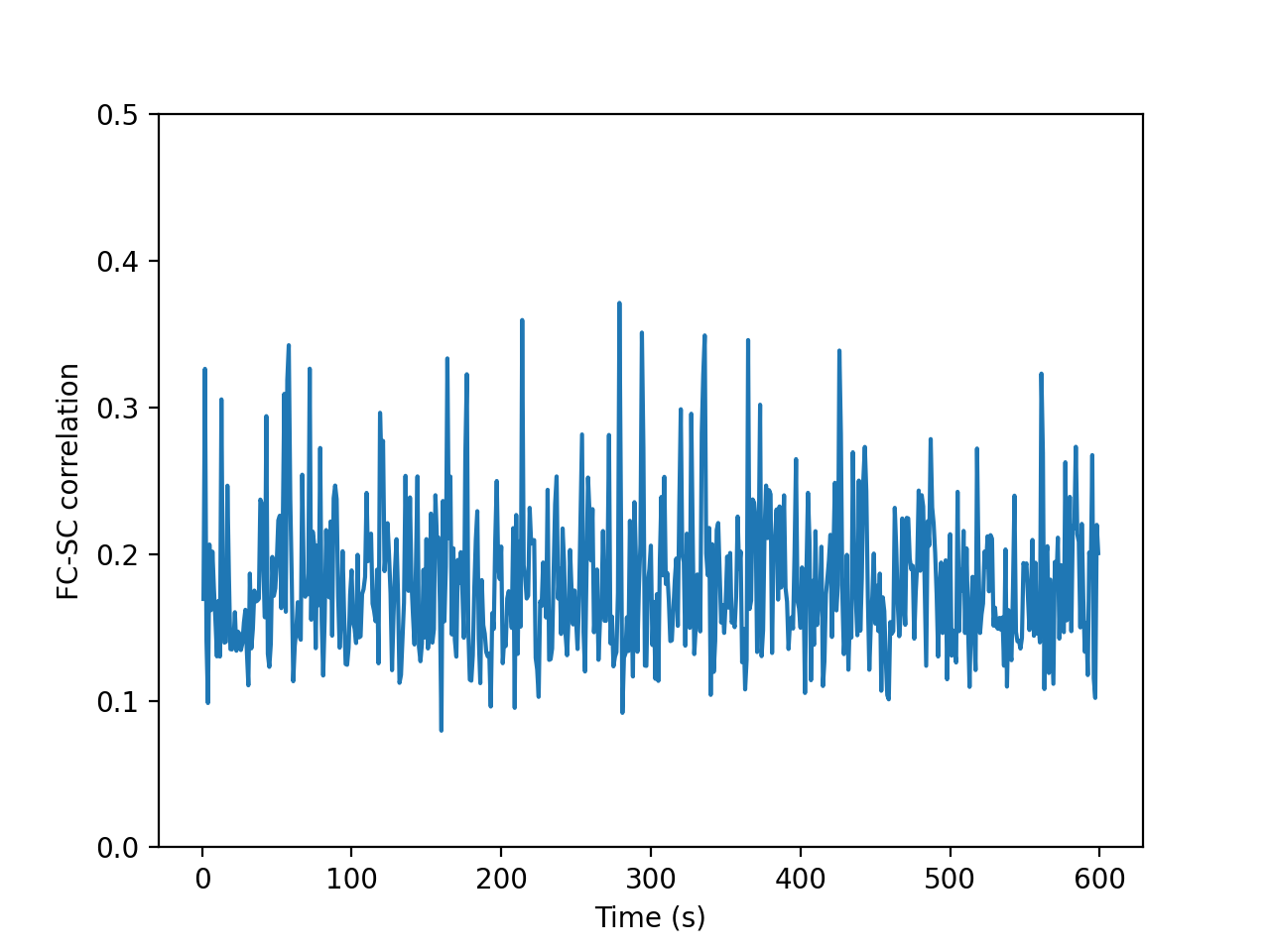In :
%pylab nbagg
import sys
from tvb.simulator.lab import *
LOG = get_logger('demo')
import scipy.stats
from sklearn.decomposition import FastICA
import time
import utils

Populating the interactive namespace from numpy and matplotlib


# Introduction¶

Fluctuations in brain activity in non-task conditions are now a well-established phenomena in the literature. These fluctuations are not random but shown to exhibit spatial patterns, referred to as resting state networks. Despite being readily identifiable during rest, these networks are related to specific functions and on the other hand abnormalities in such RSNs have been associated with pathology.

In the following, we will demonstrate some starting points for modeling resting state networks in TVB, using the default data set.

# Setting up the simulation¶

In the following, we'll use a basic region level simulation, with the generic oscillator set in an excitable regime, linear coupling with low strength, a stochastic integrator with low noise and a temporal average monitor at 200 Hz.

These settings are a good starting point for modeling resting state patterns because no particular factor dominates the dynamics and a balance between the structural connectivity, moderate intrinsic excitability and noise comes into play.

In :
def run_sim(conn, cs, D, cv=3.0, dt=0.5, simlen=1e3):
sim = simulator.Simulator(
model=models.Generic2dOscillator(a=numpy.array([0.0])),
connectivity=conn,
coupling=coupling.Linear(a=numpy.array([cs])),
monitors=(monitors.TemporalAverage(period=5.0),) # 200 Hz
)
sim.configure()
(t, y), = sim.run(simulation_length=simlen)
return t, y[:, 0, :, 0]

conn = connectivity.Connectivity.from_file()

WARNING  File 'hemispheres' not found in ZIP.


One of the common features of simulations is an initial transient, so we'll perform a one minute simulation, and as soon as the time series have been generated, we check that the transient has decayed:

In :
tic = time.time()
t, y = run_sim(conn, 6e-2, 5e-4, simlen=10*60e3)
'simulation required %0.3f seconds.' % (time.time() - tic, )

Out:
'simulation required 378.781 seconds.'

# Functional Connectivity¶

Next, to quickly assess the presence of a network structure in the time series, we window the time series into 1 second non overlapping windows, obtain per-window correlation matrices

In :
cs = []
for i in range(int(t[-1]/1e3)):
cs.append(corrcoef(y[(t>(i*1e3))*(t<(1e3*(i+1)))].T))
cs = array(cs)
cs.shape

Out:
(599, 76, 76)

The strength of correlation can be assessed statistically by Fisher Z transforming the coefficients and applying a t-test,

In :
cs_z = arctanh(cs)
for i in range(cs.shape):
cs_z[:, i, i] = 0.0
_, p = scipy.stats.ttest_1samp(cs, 0.0)

/Library/Anaconda/anaconda3/envs/tvb-run/lib/python3.7/site-packages/ipykernel_launcher.py:1: RuntimeWarning: divide by zero encountered in arctanh
"""Entry point for launching an IPython kernel.


Which we then visualize the structural connectivity (left) and functional connectivity (right) as an adjacency matrices applying a threshold on significance:

In :
figure(figsize=(10, 4))
subplot(121), imshow(conn.weights, cmap='binary', interpolation='none')
subplot(122), imshow(log10(p)*(p < 0.05), cmap='gray', interpolation='none');
show()/Library/Anaconda/anaconda3/envs/tvb-run/lib/python3.7/site-packages/ipykernel_launcher.py:3: RuntimeWarning: divide by zero encountered in log10
This is separate from the ipykernel package so we can avoid doing imports until


We can see there are significant deviations in the FC from the SC which are especially prominent in the interhemispheric FC, where interactions are found despite limited interhemispheric SC.

We can then ask what degree of similarity there is between the average functional connectivity and the structural connectivity, and how it varies over time:

In :
figure()
plot(r_[1:len(cs)+1], [corrcoef(cs_i.ravel(), conn.weights.ravel())[0, 1] for cs_i in cs])
ylim([0, 0.5])
ylabel('FC-SC correlation')
xlabel('Time (s)');
show()# Seed-region correlation maps¶

A common visualization of FC specific to a given is to pull out its row of the FC matrix and plot a colormap on the anatomy. We can do this will the simulated functional connectivity to identify which regions are highly coordinated with the seed region.

In :
ctx = cortex.Cortex.from_file()
ctx.region_mapping_data.connectivity = conn
rm = ctx.region_mapping

def plot_roi_corr_map(reg_name):
roi = list(conn.ordered_labels).index(reg_name)
cs_m = cs[2:].mean(axis=0)
utils.multiview(cs_m[roi][rm], cortex=ctx, shaded=False, suptitle=reg_name, figsize=(10, 5))


As a few examples of such maps, seeding in the left motor cortex, right ventrolateral prefront cortex, and right superior parietal cortex:

In :
for reg in 'lM1 rPFCVL rPCS'.split():
plot_roi_corr_map(reg)